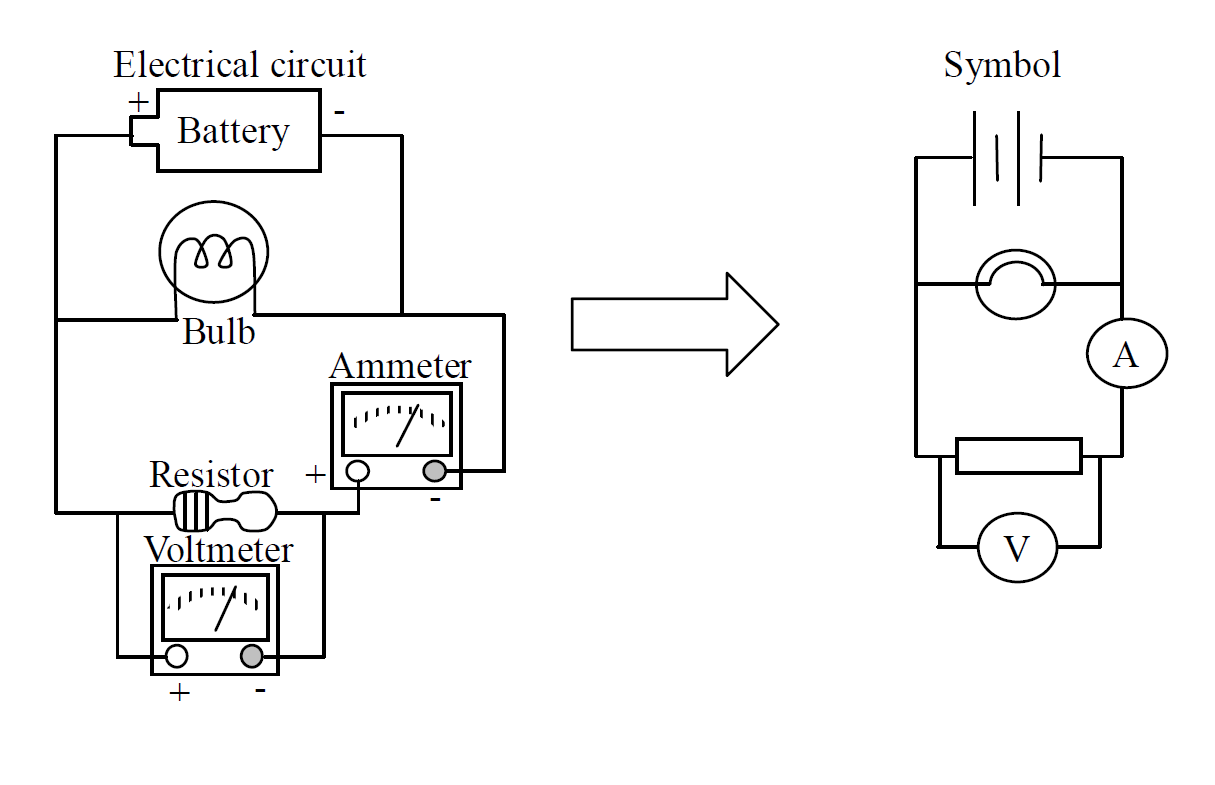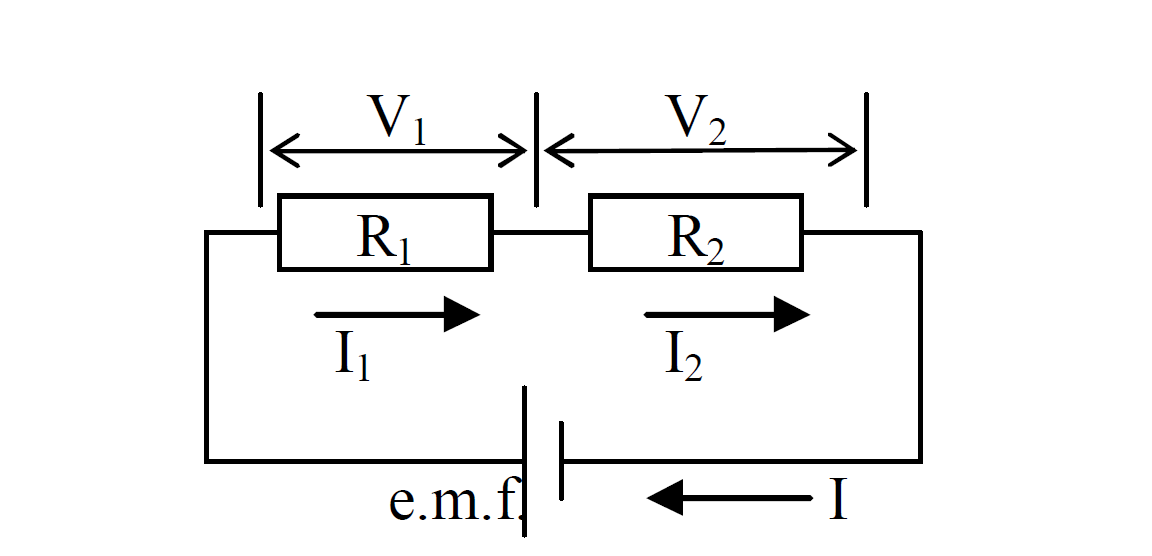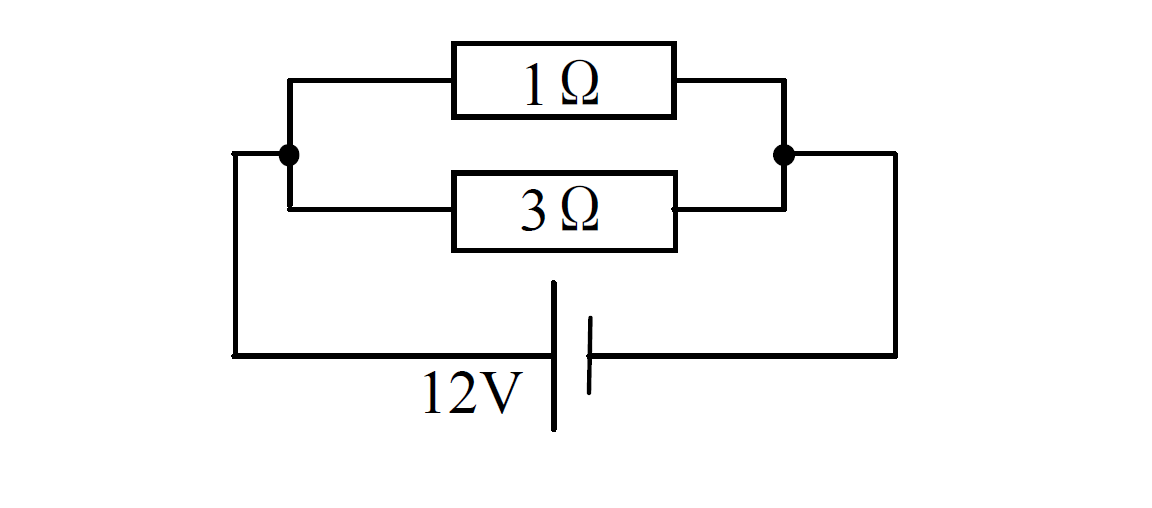# Resistance

The resistance in a circuit is the opposition to the flow of current.

The unit of resistance is Ohm [Ω].

- Some components in a circuit such as a bulb or a heater have resistance.

- A device which provides some resistance in the circuit is called a resistor

- A resisitor which can vary resistance is called the rheostat.

Formula

$R=\frac{V}{I}$

Given that

R: Resistance [Ω]

V: Potential difference [V]

I: Current [A]

This relationship is called Ohm’s law.

#### Example 1

A current of 2A flows through a conductor. The conductor has the p.d. of 12V. Find the resistance of the conductor.

Solution

$R=\frac{V}{I}$

Given that

I = 2A

V = 12V

R = ?

$R=\frac{\mathrm{12}}{2}$

Answer: $R=\mathrm{6\Omega }$

#### Example 2

Find the p.d. across a 1.5Ω resistor when a current of 4A flows through it.

Solution

$R=\frac{V}{I}$

Given that

R = 1.5Ω

I = 4A

V = ?

$\mathrm{1.5}=\frac{V}{4}$

$V=\mathrm{1.5}×4$

Answer: $V=\mathrm{6V}$

#### Example 3

Find the current flowing through a 5Ω resistor that has 20V across it.

Solution

$R=\frac{V}{I}$

Given that

R = 5Ω

V = 20V

I = ?

$5=\frac{\mathrm{20}}{I}$

$\mathrm{5I}=\mathrm{20}$

$\frac{\mathrm{5I}}{5}=\frac{\mathrm{20}}{5}$

Answer: $I=\mathrm{4A}$

#### Symbol of electrical component

When we draw a circuit, some symbols are used. The symbols comonly used are shown below.#### Example#### Series circuit

- The current is the same at all points in the series circuit.

- The sum of the p.d. V across the resistors (the total resistance) is the same as the e.m.f.

$\mathrm{e.m.f}=\mathrm{V1 + V2}=V$

= The total resistance, R, of the components connected in series circuit is equal to the sum of the separate resistances.

$R=\mathrm{R1 + R2}$#### Example

Given the circuit below, Find(a) the total resistance

(b) the p.d. of 1Ωresistor.

(c) the p.d. of 3Ω resistor.

(d) the current through 1Ω resistor.

(e) the current through 3Ω resistor.

(f) the current through the cell.

Solutions

(a)

$\frac{1}{R}=\frac{1}{R1}+\frac{1}{R2}$

Given that

R1 = 1Ω

R2 = 3Ω

R = ?

$\frac{1}{R}=\frac{1}{1}+\frac{1}{3}$

$R=\frac{3}{4}$

Answer: $R=\mathrm{0.75\Omega }$

(b)

e.m.f. = 12V

$\mathrm{e.m.f}=\mathrm{V1 + V2}=V$

Answer: $\mathrm{V1}=\mathrm{e.m.f. = 12V}$

(c)

e.m.f. = 12V

$\mathrm{e.m.f}=\mathrm{V1 + V2}=V$

Answer: $\mathrm{V2}=\mathrm{e.m.f. = 12V}$

(d)

$\mathrm{R1}=\frac{\mathrm{V1}}{I1}$

Given that

V1 = 12V

R1 = 1Ω

I1 = ?

$1=\frac{\mathrm{12}}{I1}$

Answer: $\mathrm{I1}=\mathrm{12A}$

(e)

$\mathrm{R2}=\frac{\mathrm{V2}}{I2}$

Given that

V2 = 12V

R2 = 3Ω

I2 = ?

$3=\frac{\mathrm{12}}{I2}$

$\mathrm{3I2}=\mathrm{12}$

$\frac{\mathrm{3I2}}{3}=\frac{\mathrm{12}}{3}$

Answer: $\mathrm{I2}=\mathrm{4A}$

(f)

$I=I1 + I2$

Given that

I = ?

I1 = 12A

I2 = 4A

$I=12 + 4$

Answer: $I=16A$